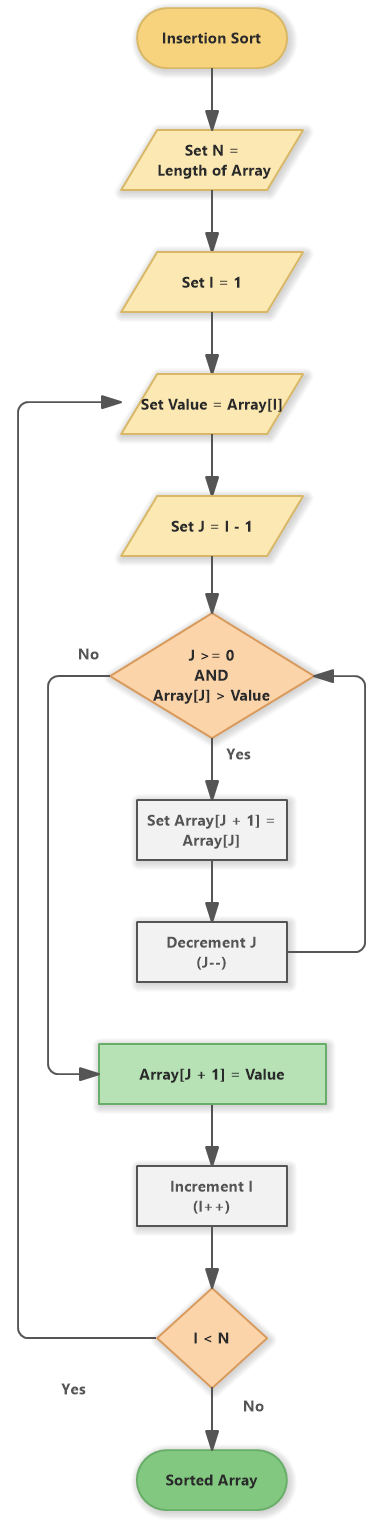# Insertion Sort (Flowchart)

Insertion sort algorithm depicted in a flowchart and pseudocode.

## Flowchart for Insertion Sort

The flowchart shows the steps of insertion sort algorithm.Insertion Sort (Flowchart)

Insertion Sort algorithm is defined by the following steps:

1. Set N = Length of Array

2. Set I = 1

3. Set Value = Array[I]

4. Set J = I - 1

5. J >= 0 AND Array[J] > Value

6. Set Array [J + 1] = Array [J]

7. Decrement J

8. J--

9. Array[J + 1] = Value

10. Increment I (I++)

11. I < N

The array is sorted.

If you are new here, you may be interested in our flowchart maker, which you can use to open the example above:

Insertion Sort (Flowchart)